# 用Python进行体育竞技分析（预测球队成绩）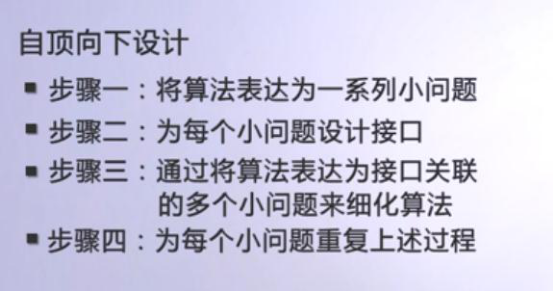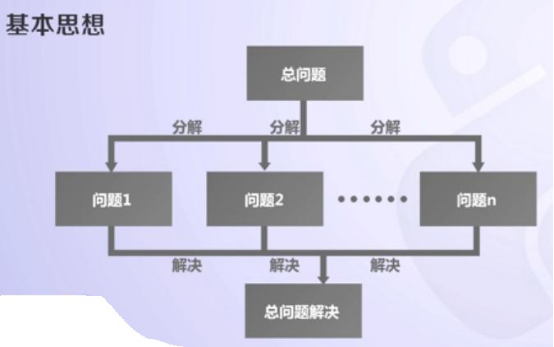`# -*- coding: utf-8 -*-"""Created on Wed May 15 12:49:17 2019@author: moyulin"""from random import randomdef printIntro(): print("BY 2018310143103") print("这个程序模拟两个选手A和B的兵乓球比赛") print("程序运行需要A和B的能力值（以0到1之间的小数表示）")def getInputs(): a = eval(input("请输入选手A的能力值(0-1): ")) b = eval(input("请输入选手B的能力值(0-1): ")) n = eval(input("请输入模拟比赛的局数: ")) return a, b, ndef simNGames(n, probA, probB): WinsA, WinsB = 0, 0 winsA, winsB = 0, 0 for i in range(1,n 1):  scoreA, scoreB = simOneGame(probA, probB)  if scoreA > scoreB:winsA  = 1  else:winsB  = 1  if i%7==0:if winsA>winsB: WinsA =1 print("单打第{}场胜利的为A".format(int(i/7)))else: WinsB =1 print("单打第{}场胜利的为B".format(int(i/7)))winsA,winsB=0,0 return WinsA, WinsBdef gameOver(a,b): if a>=10 and b>=10:  if abs(a-b)==2:return True if a<10 or b<10:  if a==11 or b==11:return True else:  return Falsedef simOneGame(probA, probB): scoreA, scoreB = 0, 0 serving = "A" while not gameOver(scoreA, scoreB):  if serving == "A":if random() < probA: scoreA  = 1else: scoreB  =1 serving="B"  else:if random() < probB: scoreB  = 1else: scoreA  = 1 serving="A"  return scoreA, scoreBdef printSummary(winsA, winsB): n = winsA   winsB print("竞技分析开始，共模拟{}场比赛".format(n)) print("选手A获胜{}场比赛，占比{:0.1%}".format(winsA, winsA/n)) print("选手B获胜{}场比赛，占比{:0.1%}".format(winsB, winsB/n))def main(): printIntro() probA, probB, n = getInputs() WinsA, WinsB = simNGames(n, probA, probB) printSummary(WinsA, WinsB)main()`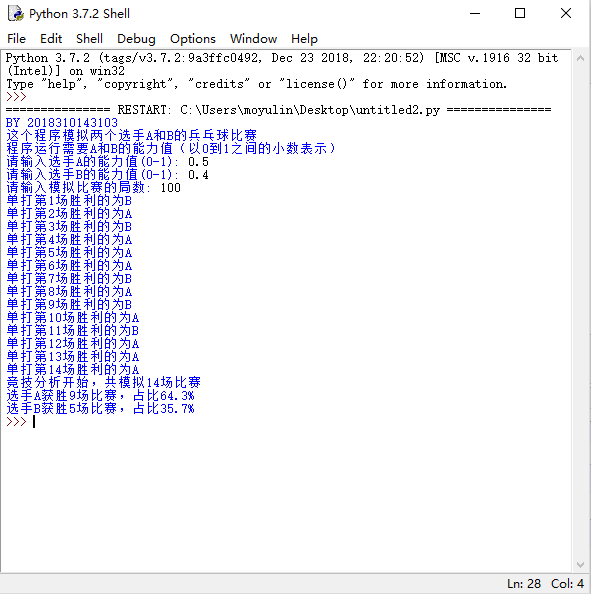win cmd打开命令行

1.安装pyinstaller库

`pip install pyinstaller`

-F,–onefile 打包一个单个文件，如果你的代码都写在一个.py文件的话，可以用这个，如果是多个.py文件就别用
-D,–onedir打包多个文件，在dist中生成很多依赖文件，适合以框架形式编写工具代码，我个人比较推荐这样，代码易于维护
-K,–tk在部署时包含TCL/TK
-a,–ascii不包含编码.在支持Unicode的python版本上默认包含所有的编码.
-d,–debug产生debug版本的可执行文件
-w,–windowed,–noconsole使用Windows子系统执行.当程序启动的时候不会打开命令行(只对Windows有效)
-c,–nowindowed,–console

2.打开命令行使用

`pyinstaller -F C:\#py文件地址`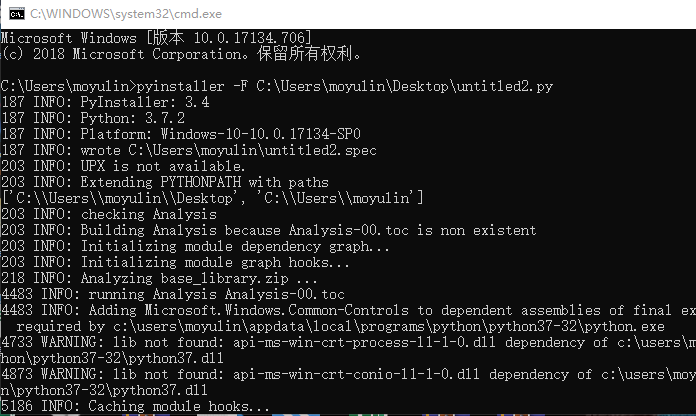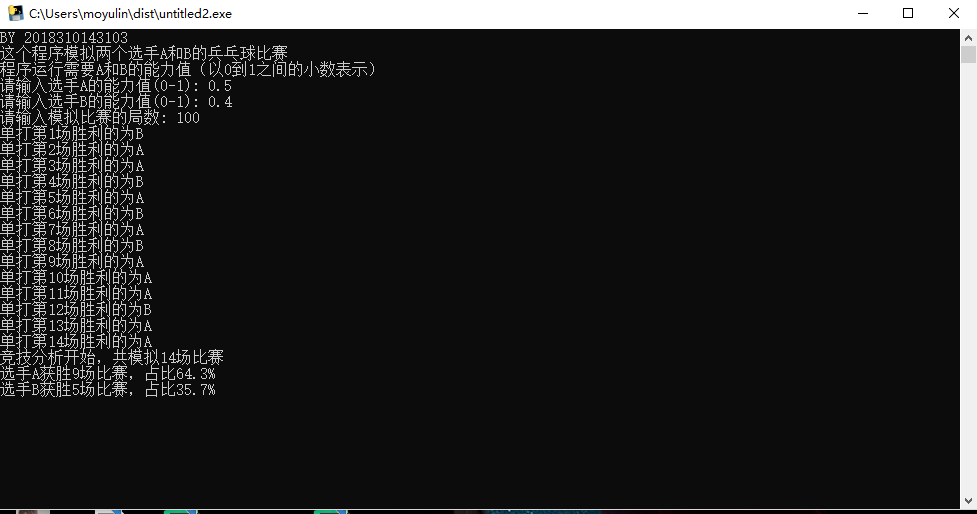`from random import randomdef printIntro(): print("by 2018310143103") print("这个程序模拟两个队A和B的篮球比赛") print("程序运行需要队A和队B的能力值（以0到1之间的小数表示）")def getInputs(): a = eval(input("请输入队A的能力值(0-1): ")) b = eval(input("请输入队B的能力值(0-1): ")) n = eval(input("模拟比赛的场次: ")) return a, b, ndef simNGames(n, probA, probB): winsA, winsB = 0, 0 for i in range(n):  scoreA, scoreB = simOneGame(probA, probB)  if scoreA > scoreB:winsA  = 1  else:winsB  = 1 return winsA, winsBdef gameOver(a,b): return a==100 or b==100def simOneGame(probA, probB): scoreA, scoreB = 0, 0 serving = "源文地址：https://www.guoxiongfei.cn/cntech/17082.html  `